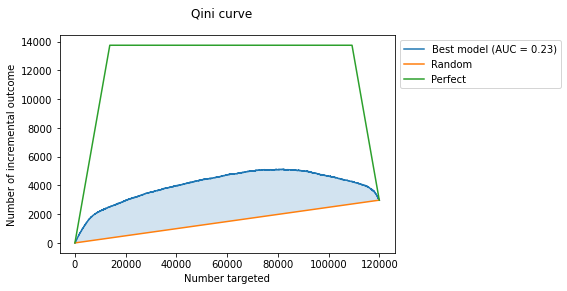MegaFon Uplift Competition¶

problem: predict the uplift by user's feature vector¶

In :
pip install scikit-uplift lightgbm -U
In :
import pandas as pd
from sklearn.model_selection import (
StratifiedShuffleSplit, GridSearchCV,
train_test_split, cross_validate, cross_val_score
)
from lightgbm import LGBMClassifier
from sklift.models import SoloModel
from sklift.viz import plot_qini_curve
from sklift.datasets import fetch_megafon
from sklift.metrics import make_uplift_scorer
In :
dataset = fetch_megafon()
data, treatment, target = dataset.data, dataset.treatment, dataset.target
data = data.set_index('id')

Out:
X_1 X_2 X_3 X_4 X_5 X_6 X_7 X_8 X_9 X_10 ... X_41 X_42 X_43 X_44 X_45 X_46 X_47 X_48 X_49 X_50
id
0 39.396577 -0.186548 19.524505 21.250208 55.291264 182.966712 -5.385606 144.573379 -12.534344 -58.279429 ... 90.877638 134.363458 -213.584582 -2.092461 -93.973258 -0.155597 -312.130733 44.798182 -125.682413 16.231365
1 38.987694 0.819522 -42.064512 -48.270949 -33.171257 179.459341 -87.151810 -162.693257 20.651652 181.635081 ... -183.840746 72.864779 559.783584 1.142391 80.037124 -1.216185 -111.473936 -127.737977 -117.501171 10.732234
2 -16.693093 1.844558 -8.615192 -18.818740 -22.271188 -116.290369 -63.816746 -38.340763 24.968496 -136.340629 ... -203.637766 2.480242 96.998504 1.100962 -33.275159 0.920926 -679.492242 -91.009397 -18.173358 14.367636
3 -72.040154 -0.226921 39.802607 16.441262 -1.112509 68.128008 23.073147 4.688858 -49.383641 -91.866107 ... 172.906875 83.951551 -323.642557 -0.369182 93.221948 -1.962380 -442.466684 -22.298302 -75.916603 11.634299
4 18.296973 0.996437 24.465307 -34.151971 24.623458 -155.455558 -12.159787 26.705778 105.864805 258.607252 ... 125.577535 -208.531112 118.902324 -0.808578 -117.497906 1.770635 627.395611 122.019189 194.091195 -11.883858

5 rows × 50 columns

In :
Out:
0      control
1      control
2    treatment
3    treatment
4    treatment
Name: treatment_group, dtype: object
In :
Out:
0    0
1    0
2    0
3    0
4    0
Name: conversion, dtype: int64

📝Solution of problem¶

In :
# make treatment binary
treatment = treatment.map({'control': 0, 'treatment': 1})

1) Conditional Independence Assumption:¶

intuition: check if treatment was random

In :
f1_micro = cross_val_score(
X=data,
y=treatment,
estimator= LGBMClassifier(random_state=42, n_jobs=-1),
scoring='f1_micro',
cv=3)

print(f'F1 micro {f1_micro.mean():.2f}')
F1 micro 0.50

Based on the user's features, the classifier makes type I and type II errors as often (50%) as it guesses the correct answer. This means that communication was carried out by random.

intuition: the model fits simultaneously on two groups with binary treatment flag as an additional user's feature. We score each object from the test sample twice: with the treatment flag equal to 1 and equal to 0. Subtracting the probabilities for each observation, we obtain an estimated uplift.

In :
# setting up data
# use test set for model evaluation after grid-search-cross-validation-tuning
# use cv set for GridSearchCV

stratify_cols = pd.concat([treatment, target], axis=1)

X_cv, X_test, y_cv, y_test, trmnt_cv, trmnt_test = train_test_split(
data,
target,
treatment,
stratify=stratify_cols,
test_size=0.2,
random_state=42
)
In :
# setting up model ...
estimator = LGBMClassifier(
random_state=42,
n_jobs=-1,
)

# ... metamodel ...
slearner = SoloModel(estimator=estimator)

# ... and uplift metric scorer to pass to cross validation
uplift_scorer = make_uplift_scorer("qini_auc_score", trmnt_cv)
In :
cv_gen = StratifiedShuffleSplit(
n_splits=3,
random_state=42
)

cross_validate(slearner,
X=X_cv,
y=y_cv,
scoring=uplift_scorer,
return_estimator=True,
cv=cv_gen,
n_jobs=-1,
fit_params={'treatment': trmnt_cv}
)
Out:
{'fit_time': array([33.87235594, 33.96732211, 33.46848416]),
'score_time': array([1.76540208, 1.70858002, 1.79931688]),
'estimator': [SoloModel(estimator=LGBMClassifier(random_state=42)),
SoloModel(estimator=LGBMClassifier(random_state=42)),
SoloModel(estimator=LGBMClassifier(random_state=42))],
'test_score': array([0.22895519, 0.22294613, 0.22625875])}
In :
grid = {
'estimator__learning_rate': [0.2],
'estimator__max_depth': 
}

grid_search = GridSearchCV(slearner,
param_grid=grid,
scoring=uplift_scorer,
n_jobs=-1,
cv=cv_gen,
return_train_score=True
)

grid_search = grid_search.fit(
X=X_cv,
y=y_cv,
treatment=trmnt_cv
)
In :
print(f"best qini score on grid search: {grid_search.best_score_:.4f}")
print(f"best params: {grid_search.best_params_}")
best qini score on grid search: 0.2269
best params: {'estimator__learning_rate': 0.2, 'estimator__max_depth': 6}
In :
slearner.set_params(**grid_search.best_params_)

slearner.fit(
X=X_cv,
y=y_cv,
treatment=trmnt_cv,
)

uplift = slearner.predict(X_test)
In :
bets_disp = plot_qini_curve(y_test, uplift, trmnt_test, perfect=True, name='Best model');
bets_disp.figure_.suptitle("Qini curve");